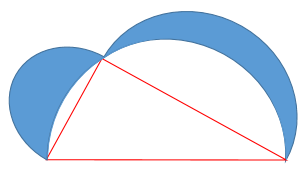Area of two crescents

Geometry Level 3Given a right triangle with area 100 square units, inscribe it inside a semicircle (hypotenuse = diameter). Then draw two semicircles using the two legs as diameters, forming two crescent shapes where the three semicircular arcs intersect. Find the combined area of the crescent shapes (shaded blue), rounded to the nearest whole number.

×